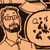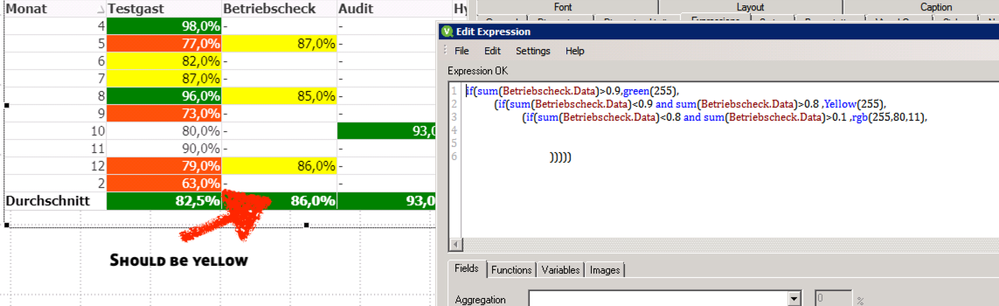# QlikView App Dev

Discussion Board for collaboration related to QlikView App Development.

Announcements
Join this live chat April 6, 10AM EST - QlikView to Qlik Sense REGISTER
cancel
Showing results for
Did you mean:Contributor III

## Dynamic Background in Totals on Straight Table

Hey Qlikkers,

i tried to build a Straight Table but the Totals Row kills me. For each Column i have an Expression how to format the Cell, but the Total Row does not really care about that. Any ideas?2 Solutions

Accepted SolutionsPartner

Hi!

Your totals are an average so you can not assign a color from a condition in Sum(Betriebscheck.Data). Try something like:

``````if(Dimensionality() = 0,
if(avg(Betriebscheck.Data)>0.9, green(255),
if(avg(Betriebscheck.Data)>0.8, Yellow(255),
if(avg(Betriebscheck.Data)>0.1, rgb(255,80,11))))
,
if(sum(Betriebscheck.Data)>0.9,green(255),
if(sum(Betriebscheck.Data)>0.8 ,Yellow(255),
if(sum(Betriebscheck.Data)>0.1, rgb(255,80,11))))
)``````

if dimensionality()=0 then it is a "total" cell. Thus, I choose a color depending on cell type: I use AVG for total cells and SUM for regular ones.

Regards,

Jaime.Anonymous
Not applicable

for showing the value in total you are using average of rows

for the background color you are only using sum(Betriebscheck_Data)! BUT the sum(Betriebscheck_Data) for total is 3,497!!! and so green is the correct color
You may use avg instead of sum for the background color.
Or you define the colors in Visual Cues for the field Betriebscheck

5 RepliesPartner

Hi!

Could you share a sample of data so we can test?

JaimeContributor III
Author

Hey Jaime,

here is a Test Version.

Thank youPartner

Hi!

Your totals are an average so you can not assign a color from a condition in Sum(Betriebscheck.Data). Try something like:

``````if(Dimensionality() = 0,
if(avg(Betriebscheck.Data)>0.9, green(255),
if(avg(Betriebscheck.Data)>0.8, Yellow(255),
if(avg(Betriebscheck.Data)>0.1, rgb(255,80,11))))
,
if(sum(Betriebscheck.Data)>0.9,green(255),
if(sum(Betriebscheck.Data)>0.8 ,Yellow(255),
if(sum(Betriebscheck.Data)>0.1, rgb(255,80,11))))
)``````

if dimensionality()=0 then it is a "total" cell. Thus, I choose a color depending on cell type: I use AVG for total cells and SUM for regular ones.

Regards,

Jaime.Anonymous
Not applicable

for showing the value in total you are using average of rows

for the background color you are only using sum(Betriebscheck_Data)! BUT the sum(Betriebscheck_Data) for total is 3,497!!! and so green is the correct color
You may use avg instead of sum for the background color.
Or you define the colors in Visual Cues for the field BetriebscheckContributor III
Author
Work's perfect. Thank you